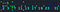# Baby steps towards test-driven development in my hobby projects

`/* * (IDE-generated boilerplate for license) *//** * (IDE-generated boilerplate for Javadoc) */public class Dashboard extends Screen implements Updateable {}`A diagram produced by Version 0.9 of my program. I added the numeric text in Photoshop Elements.
`        return (a ** ((p - 1)/2)) % p;`
`    public static byte symbolLegendre(int a, int p) {        return -2;    }`
`    @Test    public void testLegendreSymbol() {        System.out.println("Testing the Legendre symbol by way of                            quadratic reciprocity.");        byte expResult, result;        int p, q;        for (int pindex = 1; pindex < primesList.size(); pindex++) {            p = primesList.get(pindex);            for (int qindex = pindex + 1; qindex <                                      primesList.size(); qindex++) {                q = primesList.get(qindex);                expResult = symbolLegendre(q, p);                if (p % 4 == 3 && q % 4 == 3) { expResult *= -1; }                result = symbolLegendre(p, q);                assertEquals(expResult, result);            }        }    }`
`    @Test    public void testLegendreSymbolOnGivenValues() {        System.out.println("Testing the Legendre symbol on three                            specific pairs of numbers.");        assertEquals(-1, symbolLegendre(19, 7));        assertEquals(0, symbolLegendre(42, 7));        assertEquals(1, symbolLegendre(42, 19));    }`
`    public static byte symbolLegendre(int a, int p) {        int expo = (p - 1)/2;        int symbol = ((int) Math.pow(a, expo)) % p;        return (byte) symbol;    }`
`    public static byte symbolLegendre(int a, int p) {        int expo = (p - 1)/2;        int symbol = ((int) Math.pow(a, expo)) % p;        if (symbol == p - 1) {            symbol = -1;        }        return (byte) symbol;    }`
`    public static byte symbolLegendre(int a, int p) {        int expo = (p - 1)/2;        int symbol = powerMod(a, expo, p);        if (symbol == p - 1) {            symbol = -1;        }        return (byte) symbol;    }`

Written by